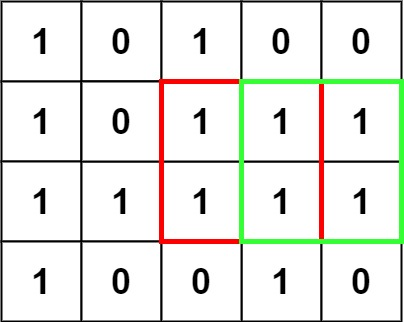# Leetcode 221 - Matrix square

Note:

• Why is it a dp question? Current sqaure might overlap with prev squares, so dp[i][j] def has some relations to dp[i-1][j-1].
• What does dp[i][j] represent?
• Make [i, j] bottom right of the square.
• How to know the deduction?
•• The side of the sqaure at dp[i][j] = 1 + min(dp[i - 1][j - 1], dp[i][j - 1], dp[i - 1][j]).
• 0 if matrix[i][j] === 0.

Question:

Given an m x n binary matrix filled with 0‘s and 1‘s, find the largest square containing only 1’s and return its area.

Example:Code:`